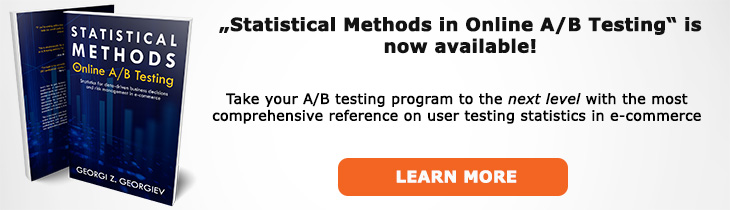# What does "Confidence Limit" mean?

Definition of Confidence Limit in the context of A/B testing (online controlled experiments).

## What is a Confidence Limit?

Aliases: confidence bound

A confidence limit is the lower or upper bound of a confidence interval (CI). It can be denoted by "LL" for lower limit and "UL" upper limit. A two-sided interval has two limits: one from below and one from above while a one-sided interval has just one limit: either a lower or upper one with the other being plus or minus infinity.

Since a confidence interval is constructed so that XX% of the time the true value of the parameter falls within the interval, the meaning of the limit once a particular interval is constructed is lost. One way to salvage it is to say, for example about a 95% one-sided interval with a lower bound, that if the true value is below the lower bound the interval would not have covered it with probability 95%. Another approach is to think about it in terms of a hypothesis test: any null hypothesis defined so that it falls entirely below the lower limit would be rejected at the 95% significance level or higher.

To take a more concrete example - if an observed lower interval limit of a 95% one-sided CI for relative difference in proportions (% lift) is at 3%, then we know that had the true lift been less than 3%, the experiment would have only produced such an outcome with probability less than or equal to 5%.

## Related A/B Testing terms

Confidence Interval

Confidence Level

Like this glossary entry? For an in-depth and comprehensive reading on A/B testing stats, check out the book "Statistical Methods in Online A/B Testing" by the author of this glossary, Georgi Georgiev.## Glossary Index by Letter

Select a letter to see all A/B testing terms starting with that letter or visit the Glossary homepage to see all.